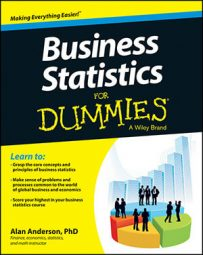Quartiles split up a data set into four equal parts, each consisting of 25 percent of the sorted values in the data set. Quartiles are related to percentiles like so:

First quartile (Q1) = 25th percentile

Second quartile (Q2) = 50th percentile

Third quartile (Q3) = 75th percentile

Because the second quartile is the 50th percentile, it's also the median of a data set. The fourth quartile usually isn't used because its value is greater than every element in a data set, so what's the point?

One commonly used approach for calculating quartiles follows these two steps:

1. Split the data into a lower half and an upper half (leaving out the median).

2. Compute the median of the lower half and the upper half.

After you've split the data into lower and upper halves, you figure out the quartiles as follows:

Q1= the median of the lower half

Q2 = the median of the entire data set

Q3 = the median of the upper half

The following data represent a sample of eight stock returns for Gamma Industries:

5, 7, 6, 3, 0, –2, 4, 3

The sorted values are:

–2, 0, 3, 3, 4, 5, 6, 7

In this example, you have eight elements. Because 8 is an even number, the median is the average of the fourth and fifth elements: 2, 0, 3, 3, 4, 5, 6, 7

(3 + 4)/2 = 3.5. Therefore, the second quartile (Q2) is 3.5.

The values below the median constitute the lower half of the sorted sample

–2, 0, 3, 3

The values above the median constitute the upper half of the sorted sample

4, 5, 6, 7

Both the lower and upper halves have four sample elements. Because 4 is an even number, the median is the average of the second and third elements.

For the lower half, the median is: (0 + 3)/2 = 1.5. This is the average value of the two middle elements. Therefore, the first quartile (Q1) is 1.5.

For the upper half, the median is (5 + 6)/2 = 5.5. Therefore, the third quartile (Q3) is 5.5.

As with percentiles, Microsoft Excel uses a different approach to computing quartiles; if you use the QUARTILE function, you will get 3.5 for Q2, but you will also get

2.25 for Q1 (instead of 1.5)

5.25 for Q3 (instead of 5.5)